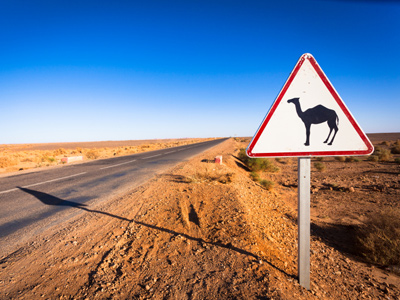This roadsign has the shape of a triangle.

# Shapes - Shape Identification

This Math quiz is called 'Shapes - Shape Identification' and it has been written by teachers to help you if you are studying the subject at middle school. Playing educational quizzes is a fabulous way to learn if you are in the 6th, 7th or 8th grade - aged 11 to 14.

It costs only \$12.50 per month to play this quiz and over 3,500 others that help you with your school work. You can subscribe on the page at Join Us

In Maths, shapes are important. Octagons and hexagons are different shapes - do you know what they look like? This quiz has a variety of two dimensional (2D) shapes that you will need to identify.

See how many you recognize by playing this quiz on the subject.

1.
Which of the shapes below is the parallelogram?
A
B
C
D
2.
Which of the shapes below is the trapezium?
A
B
C
D
3.
Which of the shapes below is a pentagon?
A
B
C
D
4.
Which shape below is a circle?
A
B
C
D
5.
From the shapes below, can you recognize the octagon?
A
B
C
D
It is in fact a regular octagon as all eight sides are of equal length and all internal angles are of the same size
6.
Which of the shapes below is a rectangle?
A
B
C
D
7.
How many of the shapes below are polygons?
1
2
3
4
A polygon has at least 3 straight sides and angles
8.
Which of the shapes below is NOT a polygon?
A
B
C
D
The circle does not have any straight sides or angles and is therefore not a polygon
9.
Can you pick out the hexagon in the picture below?
A
B
C
D
10.
Which of the shapes below is a triangle?
A
B
C
D
Author:  Frank Evans